### IMO Shortlist 2006 problem G4

Kvaliteta:
Avg: 3,0
Težina:
Avg: 7,0
Let$ABC$ be a triangle such that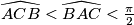$\widehat{ACB} < \widehat{BAC} < \frac {\pi}{2}$. Let$D$ be a point of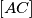$[AC]$ such that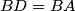$BD = BA$. The incircle of$ABC$ touches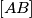$[AB]$ at$K$ and$[AC]$ at$L$. Let$J$ be the center of the incircle of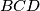$BCD$. Prove that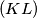$(KL)$ intersects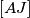$[AJ]$ at its middle.
Izvor: Međunarodna matematička olimpijada, shortlist 2006